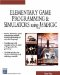# Sine

This command calculates the sine of an angle.

` x = sin(y) `

### Parameters

 x The sine of angle y . Angle y is in radians.

### Notes

Please see Chapter 5 for an explanation of the sine function. The sine is used to find the vertical speed of an object that is moving up at an angle, such as an aircraft climbing.

### Sample Code

The following code sends the angle of roll about the y-axis to the screen; this is controlled with the left and right arrow keys. The program prints the angle and sine of the angle to the screen.

` oworld = New World(); ocamera = New Camera(oworld); sphere = oworld.CreateSphere(50,50,50,25,25); ocamera.MoveBack(1200); sphere.Walk(); //Loop While(TRUE)  {   z = sphere.getyangle();   Y = sin(z);   SetText(yangle  + z + sin  + y);   } `Elementary Game Programming & Simulations Using Jamagic (Charles River Media Game Development)
ISBN: 1584502614
EAN: 2147483647
Year: 2002
Pages: 105
Authors: Sergio Perez